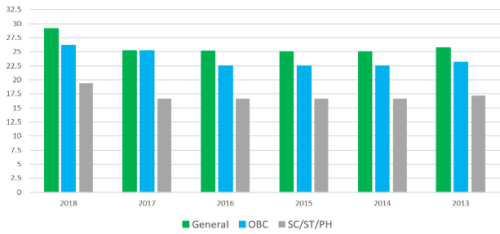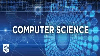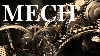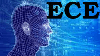Courses

# How to Prepare for Electrical Engineering GATE ? Step by Step Guide for GATE Electronics and Communication Engineering (ECE) Notes | EduRev

## Electrical Engineering (EE) : How to Prepare for Electrical Engineering GATE ? Step by Step Guide for GATE Electronics and Communication Engineering (ECE) Notes | EduRev

The document How to Prepare for Electrical Engineering GATE ? Step by Step Guide for GATE Electronics and Communication Engineering (ECE) Notes | EduRev is a part of the Electrical Engineering (EE) Course Mock Test Series for Electrical Engineering (EE) GATE 2020.
All you need of Electrical Engineering (EE) at this link: Electrical Engineering (EE)

## How to Prepare for Electrical Engineering (EE) for GATE 2020:

The Graduate Aptitude Test in Engineering (GATE) is one of the comprehensive exams to test the engineering mathematics, verbal ability and subject on the specific fields for which the students are chosen. GATE Electrical Engineering syllabus consists special and core topics. Both core and special topics are taken from your 4 year engineering study. So, you need to focus on the basics and then move on to the advanced concepts.

While preparing for Electrical Engineering part, you must have a proper plan of action in your mind as only then, you will be able to complete the syllabus and score good in this section.Electrical Engineering (EE) For GATE 2020

Includes  34 docs, 348 videos & 85 tests

## Here are a few tips and tricks for the preparation of Electric Engineering for GATE 2020:

1. Know the Syllabus and Exam Pattern:

If you are preparing for GATE Electrical Engineering (EE) 2020, you need to have a clear idea about the GATE exam pattern and syllabus for GATE EE. The exam pattern of GATE will help the candidates to know the complete scheme of examination in terms of number of questions, marks, mode of the paper, marking scheme,etc. It is important for the candidates to know their exam pattern in detail before preparing for the examination.

Also, you must go through the syllabus for GATE before starting with the preparation. The syllabus will help the candidates to understand the priority and weight age of each topic under different sections. You will get to know the topics that are already covered by you and the ones that might be new for you and need to be focused on.

2. Make and follow a Time Table:

Once you have gone through the GATE Electrical Engineering (EE) syllabus and exam pattern, you should prepare a study plan for the various topics included in Electrical engineering (EE) for GATE. Time management during preparation is really important as then, you will have enough time for every subject and no time constraints would arise. The time table would help you distribute your syllabus and study time. Follow the schedule very strictly and you will be able to cover the entire syllabus in time and have time for revision.

3. Gain Conceptual Knowledge:

You need to have a very strong fundamental knowledge of all the topics under Electrical engineering syllabus. The basic concepts will make it easier for you to understand the higher level concepts. Concepts are to be understood, not mugged up. Once you clearly see each concept for what it is all about, you will be ready for the next step. Understand the concept in such a manner that you can visualize them.

4. Good Time Management Skills:

You need a proper time management strategy during the preparation as you do not want to miss any topic just because of the lack of time. Time management applies not only to the time you take out to study but also on how you utilize those 4-5 hours every day. Learn any tricks that are there to solve certain kind of questions. This way you will be able to attempt a good number of questions in a short amount of time.

Practice as many questions as possible for Electrical engineering. Practice with subject wise tests and Chapter wise tests for Electrical Engineering (EE) for GATE. Solve GATE mock tests for EE to become perfect in the subject/topic of EE. These mock tests will help you with speed and accuracy. Take GATE EE mock tests and analyze your performance. Identify your weaknesses academically and skill-wise. You will have time to work on them and improve your performance with every mock test.

## GATE EE Practice tests

GATE Past Year Papers for Practice (All Branches)

Includes  380 docs & 97 tests

6. Attempt Previous Year Papers:

Previous year papers and Mock tests help you know the pattern of the exam and also the type of questions you can expect. Evaluate your performance regularly with these GATE EE past year papers.  So, solve as many question papers as possible and get yourself prepared for the exam. Attempt the previous year paper in a limited amount of time and check your speed and accuracy. You will get a good idea about what you need to work on to improve your performance.

## Previous Year Cut off Trends for GATE EE:

 Year General OBC SC/ST/PH 2018 29.1 26.1 19.4 2017 25.20 25.20 16.70 2016 25.1 22.5 16.7 2015 25 22.5 16.67 2014 25 22.5 16.67 2013 25.74 23.17 17.16## Recommended Books for GATE Electrical Engineering Preparation:

1. Engineering Mathematics

- Advanced Engineering Mathematics by E.Kreyszig

2. Networks

- Engineering Circuit Analysis by Hayt and Kemmerly

3. Signal and systems

- Signals and Systems by Oppenheim and Wilsky

- Signals and Systems- Nagoor Kani

4. Electrical Machine

- Electrical Machinery by P.S.Bimbhra

5. Power system

- Power Systems Engineering by Nagrath and Kothari

- Power Systems by JB Gupta & CL Wadhwa

6. Power Electronics

- For Beginners- Power Electronics by MH Rashid

- Power Electronics by P.S.Bimbhra

7. Control system

- Control Systems Engineering by Nagrath and Gopal

8. Measurement

- Electrical and Electronic Measurement and Instrumentation by AK Sawhney

9. Analog

- Electronic Devices and Circuit Theory by Boylestad

10. Digital Circuits

- For Digital Electronics, refer to Digital Design by M.Morris Mano

11. Electro Magnetics

- Mathew N. O. SADIKU-Elements of Electromagnetics

- William .H.Hayt - Engineering Electromagnetics

12. For Problem Solving

- GATE Electrical by RK Kanodia

13. Circuit analysis

- Transient Analysis Of Electric Power Circuits by Arieh L Shenkman

## GATE 2020 Syllabus for Electrical Engineering (EE):

Engineering Mathematics

• Linear Algebra: Matrix Algebra, Systems of linear equations, Eigenvalues, Eigen vectors.
• Calculus: Mean value theorems, Theorems of integral calculus, Evaluation of definite and improper integrals, Partial Derivatives, Maxima and minima, Multiple integrals, Fourier series, Vector identities, Directional derivatives, Line integral, Surface integral, Volume integral, Stokes’s theorem, Gauss’s theorem, Green’s theorem.
• Differential equations: First order equations (linear and nonlinear), Higher order linear differential equations with constant coefficients, Method of variation of parameters, Cauchy’s equation, Euler’s equation, Initial and boundary value problems, Partial Differential Equations, Method of separation of variables.
• Complex variables: Analytic functions, Cauchy’s integral theorem, Cauchy’s integral formula, Taylor series, Laurent series, Residue theorem, Solution integrals.
• Probability and Statistics: Sampling theorems, Conditional probability, Mean, Median, Mode, Standard Deviation, Random variables, Discrete and Continuous distributions, Poisson distribution, Normal distribution, Binomial distribution, Correlation analysis, Regression analysis.
• Numerical Methods: Solutions of nonlinear algebraic equations, Single and Multi‐step methods for differential equations.
• Transform Theory: Fourier Transform, Laplace Transform, z‐Transform.

Electric Circuits

Network graph, KCL, KVL, Node and Mesh analysis, Transient response of dc and ac networks, Sinusoidal steady‐state analysis, Resonance, Passive filters, Ideal current and voltage sources, The venin’s theorem, Norton’s theorem, Superposition theorem, Maximum power transfer theorem, Two‐port networks, Three phase circuits, Power and power factor in ac circuits.

Electromagnetic Fields

Coulomb's Law, Electric Field Intensity, Electric Flux Density, Gauss's Law, Divergence, Electric field and potential due to point, line, plane and spherical charge distributions, Effect of dielectric medium, Capacitance of simple configurations, Biot‐Savart’s law, Ampere’s law, Curl, Faraday’s law, Lorentz force, Inductance, Magnetomotive force, Reluctance, Magnetic circuits, Self and Mutual inductance of simple configurations.
Signals and Systems
Representation of continuous and discrete‐time signals, Shifting and scaling operations, Linear Time Invariant and Causal systems, Fourier series representation of continuous periodic signals, Sampling theorem, Applications of Fourier Transform, Laplace Transform and z-Transform.

Electrical Machines

Single phase transformer: equivalent circuit, phasor diagram, open circuit and short circuit tests, regulation and efficiency; Three phase transformers: connections, parallel operation; Auto‐transformer, Electromechanical energy conversion principles, DC machines: separately excited, series and shunt, motoring and generating mode of operation and their characteristics, starting and speed control of dc motors; Three phase induction motors: principle of operation, types, performance, torque-speed characteristics, no-load and blocked rotor tests, equivalent circuit, starting and speed control; Operating principle of single phase induction motors; Synchronous machines: cylindrical and salient pole machines, performance, regulation and parallel operation of generators, starting of synchronous motor, characteristics; Types of losses and efficiency calculations of electric machines.

Power Systems

Power generation concepts, ac and dc transmission concepts, Models and performance of transmission lines and cables, Series and shunt compensation, Electric field distribution and insulators, Distribution systems, Per‐unit quantities, Bus admittance matrix, Gauss- Seidel and Newton-Raphson load flow methods, Voltage and Frequency control, Power factor correction, Symmetrical components, Symmetrical and unsymmetrical fault analysis, Principles of over‐current, differential and distance protection; Circuit breakers, System stability concepts, Equal area criterion.

Control Systems

Mathematical modeling and representation of systems, Feedback principle, transfer function, Block diagrams and Signal flow graphs, Transient and Steady‐state analysis of linear time invariant systems, Routh-Hurwitz and Nyquist criteria, Bode plots, Root loci, Stability analysis, Lag, Lead and Lead‐Lag compensators; P, PI and PID controllers; State space model, State transition matrix.

Electrical and Electronic Measurements

Bridges and Potentiometers, Measurement of voltage, current, power, energy and power factor; Instrument transformers, Digital voltmeters and multimeters, Phase, Time and Frequency measurement; Oscilloscopes, Error analysis.

Analog and Digital Electronics

Characteristics of diodes, BJT, MOSFET; Simple diode circuits: clipping, clamping, rectifiers; Amplifiers: Biasing, Equivalent circuit and Frequency response; Oscillators and Feedback amplifiers; Operational amplifiers: Characteristics and applications; Simple active filters, VCOs and Timers, Combinational and Sequential logic circuits, Multiplexer, Demultiplexer, Schmitt trigger, Sample and hold circuits, A/D and D/A converters, 8085Microprocessor: Architecture, Programming and Interfacing.

Power Electronics

Characteristics of semiconductor power devices- Diode, Thyristor, Triac, GTO, MOSFET, IGBT; DC to DC conversion: Buck, Boost and Buck-Boost converters; Single and three phase configuration of uncontrolled rectifiers, Line commutated thyristor based converters, Bidirectional ac to dc voltage source converters, Issues of line current harmonics, Power factor, Distortion factor of ac to dc converters, Single phase and three phase inverters, Sinusoidal pulse width modulation.

## Similarly, Prepare For Other Subjects Of Gate Here:

How to prepare for GATE Civil Engineering?

How to prepare for GATE Electronics and communication Engineering?

How to prepare for GATE Computer Science Engineering(CSE)?

How to prepare for GATE Mechanical Engineering?

## Comprehensive Courses for All Different Streams of Engineering:GATE Past Year Papers for Practice (All Branches)

Includes  380 docs & 97 testsGeneral Aptitude for GATE 2020

Includes  10 docs, 17 videos & 33 testsComputer Science Engineering (CE) For GATE 2020

Includes  127 docs, 196 videos & 103 testsMechanical Engineering (ME) for GATE 2020

Includes  40 docs, 222 videos & 192 testsElectronics & Communication Engineering (EC) For GATE 2020

Includes  37 docs, 222 videos & 108 testsCivil Engineering (CE) For GATE 2020

Includes  26 docs & 41 testsHow to prepare for GATE: Preparation Strategy

Includes 11 videos

Offer running on EduRev: Apply code STAYHOME200 to get INR 200 off on our premium plan EduRev Infinity!

## Mock Test Series for Electrical Engineering (EE) GATE 2020

40 docs|191 tests

,

,

,

,

,

,

,

,

,

,

,

,

,

,

,

,

,

,

,

,

,

;